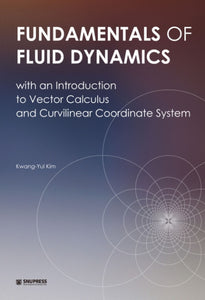Seoul National University Press

# Fundamentals of Fluid Dynamics with an Introduction to Vector Calculus and Curvilinear Coordinate System

Regular price \$38.00 Sale price \$65.45
Shipping calculated at checkout.

ISBN: 9788952116253

This book is an introduction to fundamentals of fluid dynamics and is intended for undergraduate students and also low-level graduate students. The amount of materials in the book is reasonable to cover in one semester. An instructor can choose to skip some parts of the book according to her/his class schedules and the level of students taking this course. Many figures and illustrations are included to facilitate firmer grasp of the physical concepts and the mathematical details addressed. Each chapter contains a number of solved problems. The enclosed solutions are very detailed, in general, so that students understand how each problem is approached. More problems with solutions will be added to the website of this book for instructors.

Kwang-Yul Kim

Prof. Kwang-Yul Kim received a Ph.D. in physical oceanography from Texas A&M University in 1986.
He taught meteorology and oceanography at Texas A&M University and Florida State University until
he came back to Seoul National University in 2009 as a professor in the School of Earth and Environmental
Sciences. His notable research career includes more than 100 publications in major SCI journals
with over 1,500 citations and H index of 23. He is a member of Phi Kappa Phi Honor Society.

Contents

Preface v
Chapter 1. Vector Calculus 1
1.1. Review / 2
1.2. The Del (∇)Operator / 3
1.3. Indicial Notation / 4
1.4. The ε–δ Theorem / 7
1.5. Vector Calculus / 8
1.6. Curvilinear Coordinate System / 10
1.7. Integral Theorems / 15
Exercise Problems for Chapter 1 / 20
Solved Problems for Chapter 1 / 22
Chapter 2. Thermodynamic Properties of Fluids 35
2.1. Equation of State / 36
2.2. The First Law of Thermodynamics / 37
2.3. Isentropic Processes / 43
2.4. Compressibility / 48
Exercise Problems for Chapter 2 / 49
Solved Problems for Chapter 2 / 51
Chapter 3. Fluid Statics 63
3.1. Pressure / 64
3.2. Equation of Fluid Statics / 65
3.3. Examples of Hydrostatics / 67
3.4. Hypsometric Equation / 70
3.5. Stability of the Atmospheric Column / 73
Exercise Problems for Chapter 3 / 78
Solved Problems for Chapter 3 / 81
Chapter 4. Fluid Kinematics 97
4.1. Pictorial Method of Description / 98
4.2. Eulerian Representation / 98
4.3. Particle Derivative of the Velocity Vector / 103
4.4. Physical Meaning of Gradient, Divergence and Curl / 106
4.5. Linear and Shear Strain Rates / 110
Exercise Problems for Chapter 4 / 113
Solved Problems for Chapter 4 / 116
Chapter 5. Conservation Laws 131
5.1. Equation of Continuity / 132
5.2. Mass Conservation for Material Volume / 134
5.3. Conservation of Momentum / 135
5.4. Conservation of Vorticity / 137
Exercise Problems for Chapter 5 / 139
Solved Problems for Chapter 5 / 142
Chapter 6. Frictionless Flow 151
6.1. Force / 152
6.2. Equation of Motion / 153
6.3. Euler’s Equation / 155
6.4. Bernoulli Equation / 158
6.5. Equation of Motion in Cylindrical Coordinates / 162
Exercise Problems for Chapter 6 / 166
Solved Problems for Chapter 6 / 169
Chapter 7. Motion with Rotation 181
7.1. Vorticity / 182
7.2. Vortex Motions / 182
7.3. Circulation Theorem / 185
7.4. Vorticity Equation / 187
7.5. Vorticity Equation in Cylindrical Coordinates / 190
Exercise Problems for Chapter 7 / 194
Solved Problems for Chapter 7 / 197
Chapter 8. Velocity Potential and Stream Function 215
8.1. Velocity Potential / 216
8.2. Stream Function / 219
8.3. Vortex Filament and Vortex Tube / 224
8.4. Superposition of Flows / 226
8.5. Complex Potential / 231
Exercise Problems for Chapter 8 / 236
Solved Problems for Chapter 8 / 239
Chapter 9. Viscous Flow 253
9.1. Physical Meaning of Viscous Stress / 254
9.2. Symmetry of Viscous Stress / 255
9.3. Constitutive Equation for a Newtonian Fluid / 257
9.4. Navier-Stokes Equation / 261
9.5. Simple Examples of Viscous Flow / 262
9.6. The Reynolds Number / 269
Exercise Problems for Chapter 9 / 271
Solved Problems for Chapter 9 / 274
Chapter 10. The Effect of the Earth’s Rotation 295
10.1. Motion in a Rotating Frame of Reference / 296
10.2. Fictitious Acceleration in a Rotating Frame / 297
10.3. Equation of Motion in a Rotating Frame / 299
10.4. Vorticity Equation in a Rotating Frame / 300
10.5. The Geostrophic Equation / 304
10.6. Vorticity Conservation on the β-Plane / 306
10.7. The Rossby Number / 309
10.8. The Ekman Number / 310
Exercise Problems for Chapter 10 / 312
Solved Problems for Chapter 10 / 316
Chapter 11. Energy Conservation Principles 333
11.1. Conservation of Mechanical Energy / 334
11.2. Conservation of Internal Energy / 336
11.3. General Relations among Physical Properties / 338
11.4. Entropy Equation and the Second Law / 339
Exercise Problems for Chapter 11 / 342
Solved Problems for Chapter 11 / 345
Appendix 355
A.1. Useful Constants and Parameters / 356
A.2. MKS Units of Physical Quantities / 358
A.3. Equation of Motion in Spherical Coordinates / 359
A.4. Vorticity Equation in Spherical Coordinates /360
/Index 363